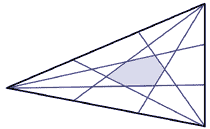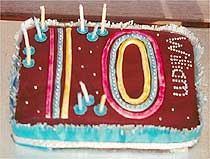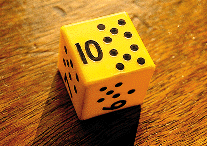# 10

= 2 × 5

= 1 + 2 + 3 + 4
A triangular number.

= 1 + 3 + 6
A tetrahedral number - the sum of the first three triangular numbers.

If a number ends with a zero it is exactly divisible by ten.

We have ten digits on our hands, and ten is the base of our number system: the decimal system. The Roman symbol for ten is X, perhaps representing two crossed hands.

The ten pins in a bowling alley are arranged in a triangular pattern.

Virgins, according to the Bible, come in tens: five foolish and five wise.

A dime is a ten cent piece in the USA.

Deca- means ten. So a decade is ten years, a decagon has ten sides and a crab is a decapod because it has ten feet. The Decalogue is a name for the biblical Ten Commandments.

A tithe means a tenth. It was the levy imposed by the church of one tenth of the produce of land and stock.

Men's lacrosse is played with teams of ten players.

Metric measurements use units in multiples of ten. In this context deca- means ten times so ten metres equals one decametre. Deca- is sometimes abbreviated to da. Deci- means one tenth so ten decimetres make a metre and ten decilitres make a litre. Deci- is sometimes abbreviated to d.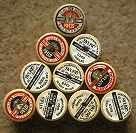Ten as a triangular number. Can you figure out what the next triangular number is?

Under English law, when you reach the age of ten –

• you can be convicted of a criminal offence if it is proved you knew that what you were doing was wrong (in Scotland this is true from age eight),
• you could be detained 'during Her Majesty's pleasure' for a specific period, including a life sentence, if you are guilty of homicide (murder).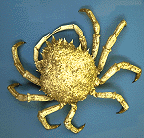Crabs and other crustaceans like shrimps and lobsters have ten legs.

An A10 sheet of paper is not much bigger than a postage stamp. It is one of the 'A' series of paper sizes. These are cleverly chosen so that the next size can always be made by folding the previous size in half, with the proportions remaining the same.

The largest size is A0 which measures 841 × 1189mm and has an area of one square metre. It is the size of a large poster. All the other sizes can be easily cut from this without any wastage –

A1 paper measures 595 × 841mm
A2 paper measures 421 × 595mm
A3 paper measures 297 × 421mm
A4 paper measures 210 × 297mm
A5 paper measures 148 × 210mm
A6 paper measures 105 × 148mm
A7 paper measures 74 × 105mm
A8 paper measures 52 × 74mm
A9 paper measures 37 × 52mm
A10 paper measures 26 × 37mm

Every page has the same width to height ratio which is 1 : 1.4142. The number 1.4142... is called the square root of 2. This means that if you multiply 1.4142... by itself on a calculator you will get an answer very close to 2.

How many A10 sheets can be cut from one A0 sheet of paper?A passion flower (Passiflora caerulea) has three-, five- and ten-fold symmetry. It has three stigmas, five stamens and ten petals.

Marion Walter is a professor of mathematics who has a special interest in the way mathematics is taught. While exploring dynamic geometry software, she discovered a new theorem which has been named Marion Walter's Theorem.

Take any triangle and divide each side into three equal parts. Draw lines from these to the opposite corners. A new shape appears in the middle of the triangle - coloured blue in the illustration below. The theorem states that the area of this shape is exactly one tenth of the area of the whole triangle. This works whatever shape of triangle you start with.